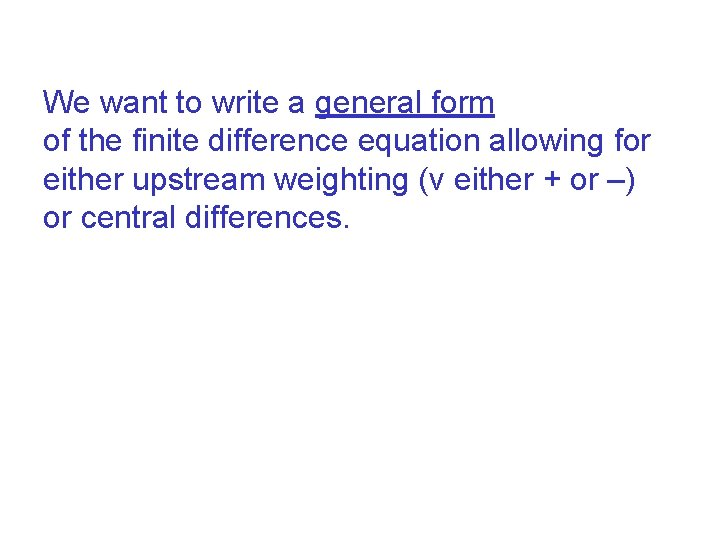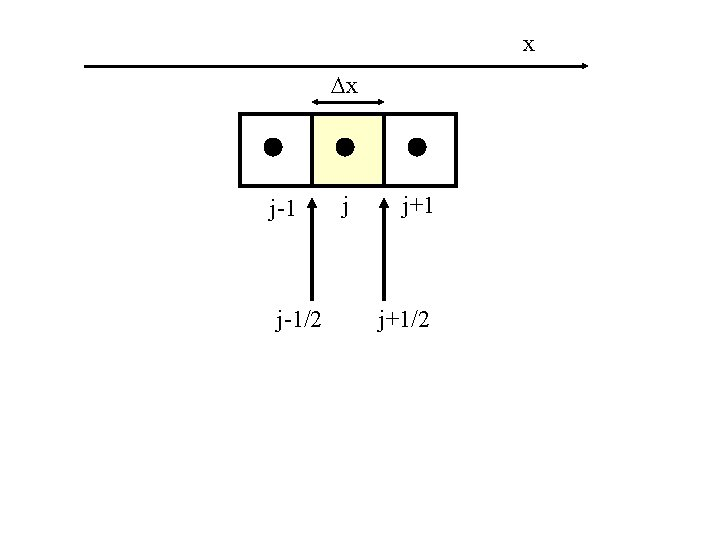# Finite Difference Solutions to the ADE Simplest form

• Slides: 19Finite Difference Solutions to the ADE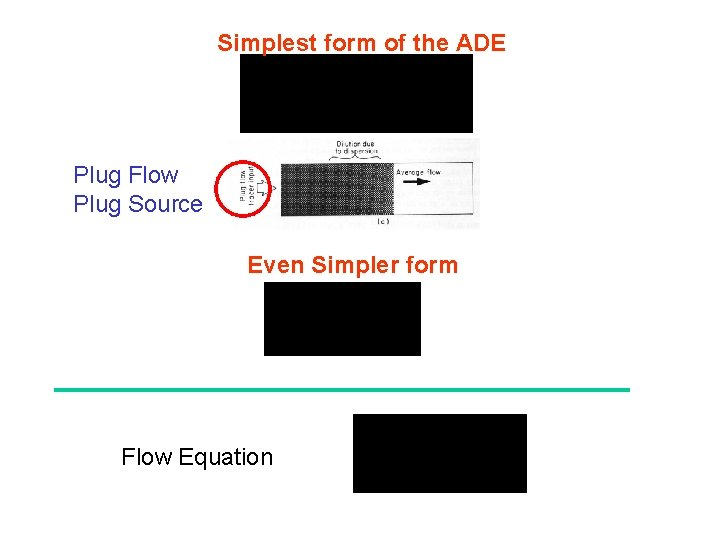Simplest form of the ADE Plug Flow Plug Source Even Simpler form Flow EquationEffect of Numerical Errors (overshoot) (MT 3 DMS manual)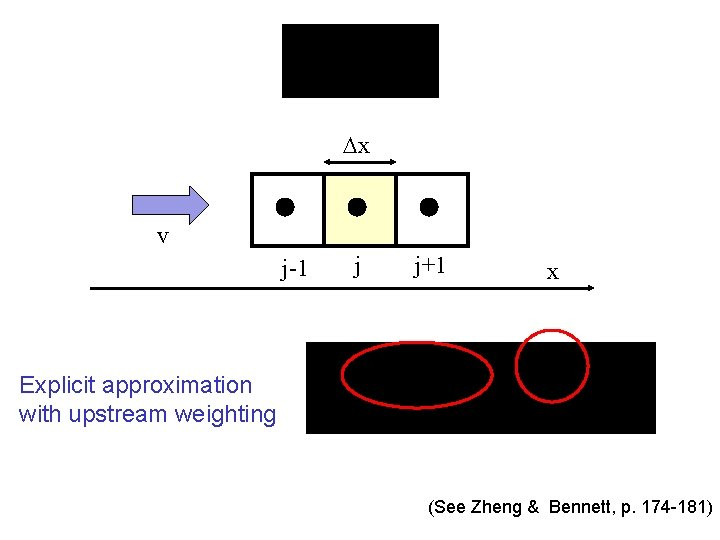x v j-1 j j+1 x Explicit approximation with upstream weighting (See Zheng & Bennett, p. 174 -181)x v j-1 j j+1 x Explicit; Upstream weighting (See Zheng & Bennett, p. 174 -181)Example from Zheng &Bennett v = 100 cm/h l = 100 cm C 1= 100 mg/l C 2= 10 mg/l With no dispersion, breakthrough occurs at t = v/ l = 1 hour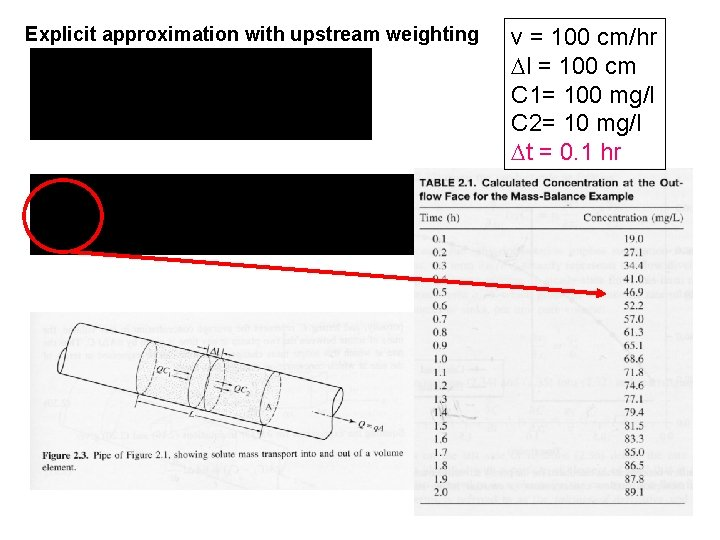Explicit approximation with upstream weighting v = 100 cm/hr l = 100 cm C 1= 100 mg/l C 2= 10 mg/l t = 0. 1 hr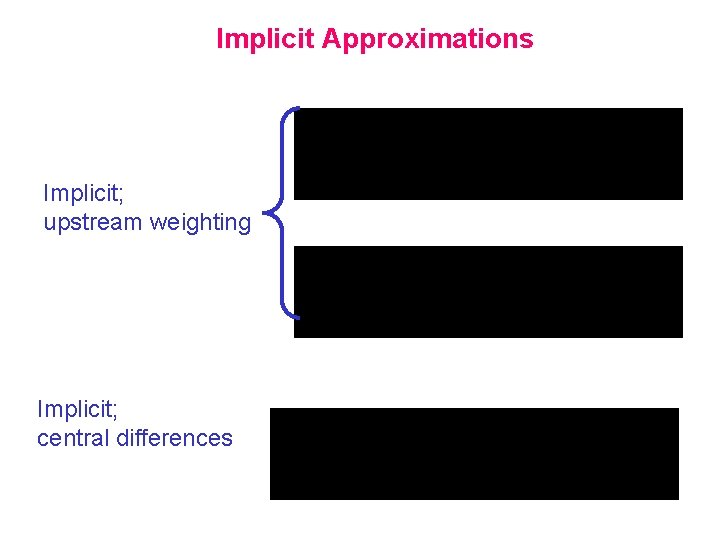Implicit Approximations Implicit; upstream weighting Implicit; central differences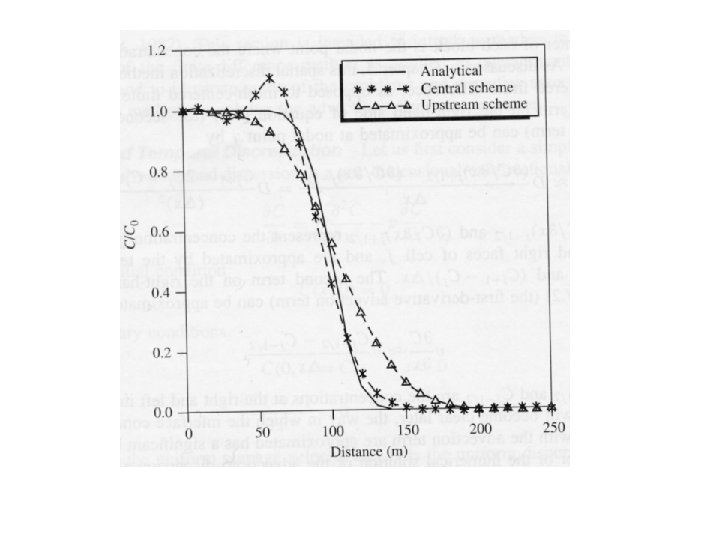= Finite Element MethodGoverning Equation for Ogata and Banks solutionx x j-1/2 j j+1/2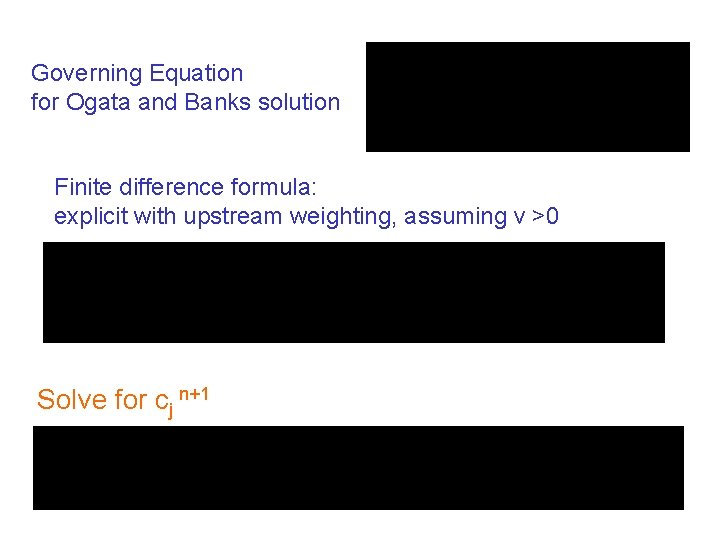Governing Equation for Ogata and Banks solution Finite difference formula: explicit with upstream weighting, assuming v >0 Solve for cj n+1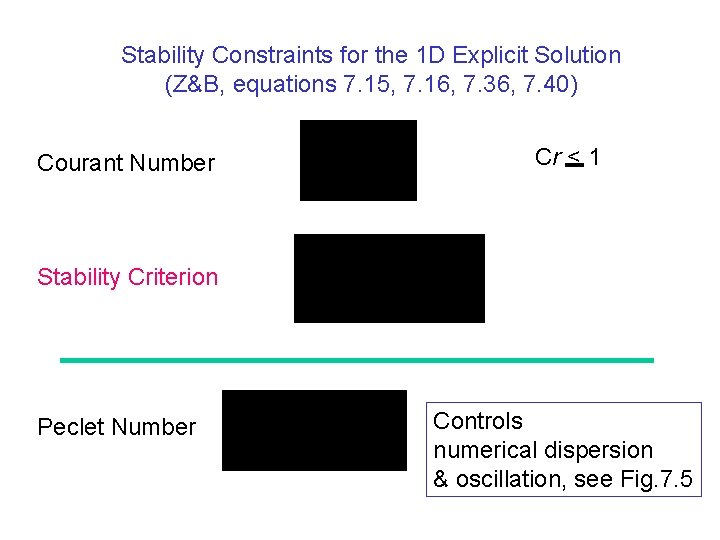Stability Constraints for the 1 D Explicit Solution (Z&B, equations 7. 15, 7. 16, 7. 36, 7. 40) Courant Number Cr < 1 Stability Criterion Peclet Number Controls numerical dispersion & oscillation, see Fig. 7. 5Boundary Conditions Specified Co concentration boundary C b= C o j-1 a “free mass outflow” boundary (Z&B, p. 285) j j+1 j-1 j C b= C j j+1